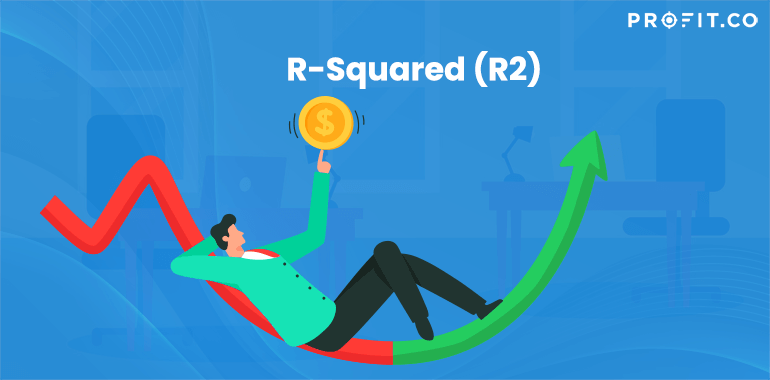Category: Finance KPIs.

Also known as the coefficient of determination, r-squared is the statistical measurement that comes from the correlation made between the performance of a certain investment and a specific benchmark index. Basically, r-squared shows what portfolio performance or degree of stock can be attributed to a certain benchmark index.

Used especially when comparing models, r-squared is a linear regression that determines how well a line fits a certain data set of observations.

Moreover, it shows the total variation in y, as a fraction, which is captured by a given model – or, in short, how well a line follows the variations that are contained by a set of data.

Things You Need to Know about R-Squared

R-squared is mainly used by investors. They use this coefficient in order to compare the performance of a portfolio with the broader market out there, and therefore, predict the trends that might occur in the (near) future.

For example, if an investor wants to buy an investment fund that has a strong correlation with the S&P 500, he or she would look for an r-squared value that’s as close as possible to 1. Why?

Because the closer the r-squared value of that investment fund is to 1, the more correlated the fund is. R-squared is also used in stocks, hedge funds, and mutual fund performance. When it comes to stocks, their movements are determined by the r-squared value. For example, it the R2 is close to one, it means that some of the stocks’ movement can be explained by the movement of the markets.

However, if the R2 value of a stock is close to zero, it means that the given stock has an independent movement when compared to the broader market.

In terms of hedge funds, the R2 is used to determine the risk that is associated with some of the factors – including the risk models of the funds. For mutual fund performance, R2 is used mainly by investors to determine the correlation between the movement of a certain fund and a benchmark index – in this case, R2 is a historical measure.

The Formula of R-Squared

While the explanation given for R-Square seems a bit complex, the formula to calculate this coefficient is quite simple. First, you have to divide the First Sum of Errors by the Second Sum of Errors, after which you subtract the final result from 1.

However, this equation is only the last step when calculating the R2 of a set data point. First of all, you have to use the line of the best-fit equation in order to predict the values on the chart that’s based on the corresponding x values.

After the line of best fit is in place, the analysts can safely come up with an error squared equation, thus keeping any errors within a relevant range.

In the end, when you have completed the list of errors, you add them up and then apply the R-Squared formula on them.

Conclusion

Basically, R-Squared is a type of statistic that can offer some information about the goodness of fit of a given model.

The coefficient of determination is a statistical measure and it shows how well and if the regression predictions are close to the real data points. Naturally, an R-Squared value that’s equal to 1 means that the predictions fit the data perfectly.

## Related Articles

• ### Sharpe Ratio

In order to be sound in your financial goals, you need to have a great source of knowledge on every... Read more